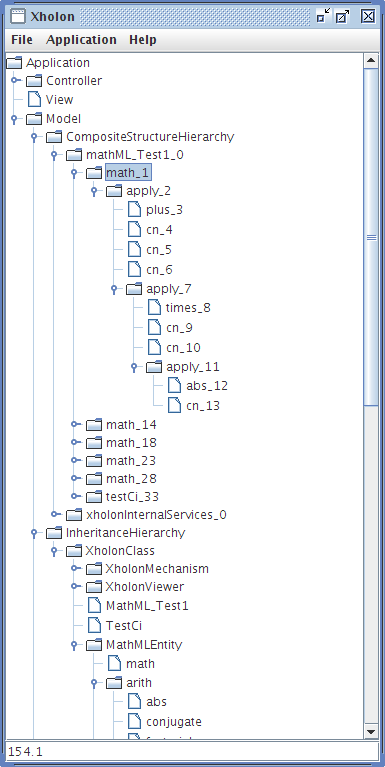### MathML

Ken Webb 2010-03-01T19:43:09Z

Mathematical Markup Language (MathML) is "an application of XML for describing mathematical notations and capturing both its structure and content." Mathematical expressions are defined as hierarchical structures. For example, the following is the MathML content for 5.1 + 2.0 + 3 + (8 * 9 * abs(-2)) :

```[itex]
<apply>
<plus/>
<cn>5.1</cn>
<cn>2.0f</cn>
<cn>3</cn>
<apply>
<times/>
<cn>8</cn>
<cn>9</cn>
<apply>
<abs/>
<cn>-2</cn>
</apply>
</apply>
</apply>
[/itex]
```

Xholon supports the MathML/XML notation. The above MathML content can be included within a Xholon application, exactly the way it's written above. The MathML mechanism in Xholon is able to execute the above expression, and calculate the answer as 154.1 .